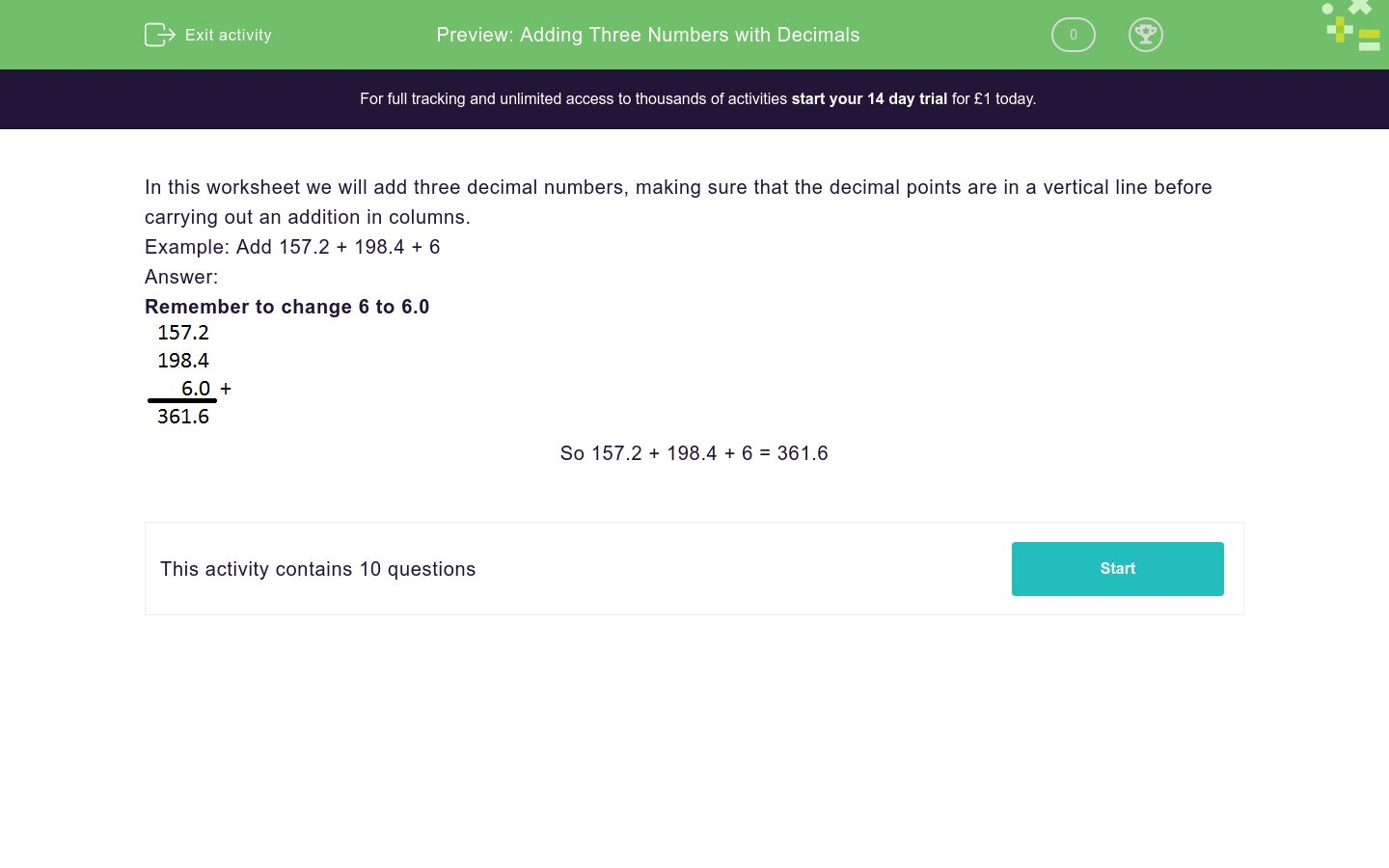# Adding Three Numbers with Decimals

In this worksheet, students add together three numbers, some of which have decimals, using columns and lining up decimals points.Key stage:  KS 2

Curriculum topic:   Number: Fractions, Decimals and Percentages

Curriculum subtopic:   Solve Problems with Decimal Places

Difficulty level:### QUESTION 1 of 10

In this worksheet we will add three decimal numbers, making sure that the decimal points are in a vertical line before carrying out an addition in columns.

Example: Add 157.2 + 198.4 + 6

Remember to change 6 to 6.0So 157.2 + 198.4 + 6 = 361.6

Find the total of

157.2 + 198.4 + 24.9 = _______

Find the total of

157.2 + 6 + 24.9 = _______

Find the total of

198.4 + 6 + 24.9 = _______

Find the total of

157.2 + 198.4 + 99.3 =________

Find the total of

157.2 + 6 + 99.3 = ________

Find the total of

198.4 + 6 + 99.3 = _________

Find the total of

157.2 + 24.9 + 99.3 = ________

Find the total of

198.4 + 24.9 + 99.3 = _______

Find the total of

6 + 24.9 + 99.3 = _______

Find the total of

33.1 + 159.3 + 52 = ________

• Question 1

Find the total of

157.2 + 198.4 + 24.9 = _______

380.5
EDDIE SAYS
Be sure to line up the decimals when adding to check if the answers are correct.
• Question 2

Find the total of

157.2 + 6 + 24.9 = _______

188.1
EDDIE SAYS
Be sure to line up the decimals when adding to check if the answers are correct.
• Question 3

Find the total of

198.4 + 6 + 24.9 = _______

229.3
EDDIE SAYS
Be sure to line up the decimals when adding to check if the answers are correct.
• Question 4

Find the total of

157.2 + 198.4 + 99.3 =________

454.9
EDDIE SAYS
Be sure to line up the decimals when adding to check if the answers are correct.
• Question 5

Find the total of

157.2 + 6 + 99.3 = ________

262.5
EDDIE SAYS
Be sure to line up the decimals when adding to check if the answers are correct.
• Question 6

Find the total of

198.4 + 6 + 99.3 = _________

303.7
EDDIE SAYS
Be sure to line up the decimals when adding to check if the answers are correct.
• Question 7

Find the total of

157.2 + 24.9 + 99.3 = ________

281.4
EDDIE SAYS
Be sure to line up the decimals when adding to check if the answers are correct.
• Question 8

Find the total of

198.4 + 24.9 + 99.3 = _______

322.6
EDDIE SAYS
Be sure to line up the decimals when adding to check if the answers are correct.
• Question 9

Find the total of

6 + 24.9 + 99.3 = _______

130.2
EDDIE SAYS
Be sure to line up the decimals when adding to check if the answers are correct. Remember to write 6.0 when adding.
• Question 10

Find the total of

33.1 + 159.3 + 52 = ________

244.4
EDDIE SAYS
Remember to write 52.0 when adding. Be sure to line up the decimals when adding to check if the answers are correct.
---- OR ----